# Principal Component Analysis and Linear Discriminant Analysis with GNU Octave

This page will show you how to do a Principal Component and Linear Discriminant Analysis with GNU Octave, a high-level language and environment for numerical computing.

## Experiment 1

### Data

Start GNU Octave from command line with:

```\$ octave
```

and create some data in `X` with corresponding classes in `c`:

```X = [2 3;3 4;4 5;5 6;5 7;2 1;3 2;4 2;4 3;6 4;7 6];
c = [  1;  1;  1;  1;  1;  2;  2;  2;  2;  2;  2];
```

Find the indices of each class {1,2}:

```c1 = X(find(c==1),:)
c2 = X(find(c==2),:)
```

And then plot the data:

```figure;

p1 = plot(c1(:,1), c1(:,2), "ro", "markersize",10, "linewidth", 3); hold on;
p2 = plot(c2(:,1), c2(:,2), "go", "markersize",10, "linewidth", 3)

xlim([0 8])
ylim([0 8])
```

We can see that both classes can clearly be separated in one dimension: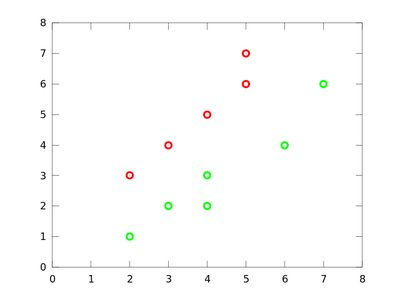So how does a Principal Component Analysis perform on this dataset?

### Principal Component Analysis

A good start for learning about a Principal Component Analysis is the Wikipedia article on Principle Component Analysis.

#### Finding the Principal Components

The math in the recommended documents can be reduced to these four lines of code:

```mu = mean(X)
Xm = bsxfun(@minus, X, mu)
C = cov(Xm)
[V,D] = eig(C)
```

That's it! Note that the Eigenvectors in GNU Octave are sorted ascending, so the last column is the first principal component. Strange to work with? So better sort the eigenvectors in a descending order:

```% sort eigenvectors desc
[D, i] = sort(diag(D), 'descend');
V = V(:,i);
```

Because all variables in this example are measured on the same scale we don't need to apply any standardization. Plot both eigenvectors:

```scale = 5
pc1 = line([mu(1) - scale * V(1,1) mu(1) + scale * V(1,1)], [mu(2) - scale * V(2,1) mu(2) + scale * V(2,1)]);
pc2 = line([mu(1) - scale * V(1,2) mu(1) + scale * V(1,2)], [mu(2) - scale * V(2,2) mu(2) + scale * V(2,2)]);

set(pc1, 'color', [1 0 0], "linestyle", "--")
set(pc2, 'color', [0 1 0], "linestyle", "--")
```

It yields a new coordinate system with the mean as origin and the orthogonal principal components as axes:According to the PCA we can safely discard the second component, because the first principal component is responsible for 85% of the total variance.

```octave> cumsum(D) / sum(D)
ans =

0.85471
1.00000
```

But what will happen if we project the data on the first principal component (red)? Do you already see the problem?

#### projection and reconstruction

To understand why the PCA fails in some situations, project the data onto the first principal component:

```% project on pc1
z = Xm*V(:,1)
% and reconstruct it
p = z*V(:,1)'
p = bsxfun(@plus, p, mu)
```

and visualize it:

```% delete old plots
delete(p1);delete(p2);

y1 = p(find(c==1),:)
y2 = p(find(c==2),:)

p1 = plot(y1(:,1),y1(:,2),"ro", "markersize", 10, "linewidth", 3);
p2 = plot(y2(:,1), y2(:,2),"go", "markersize", 10, "linewidth", 3);
```

... et voilà. The data isn't linearly separable anymore in this lower-dimensional representation.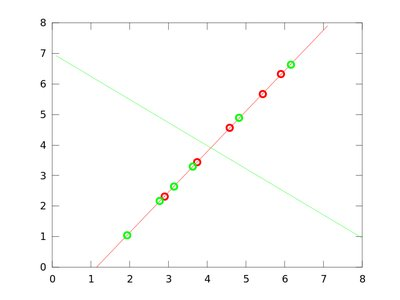... while a projection on the second principal component yields a much better representation for classification: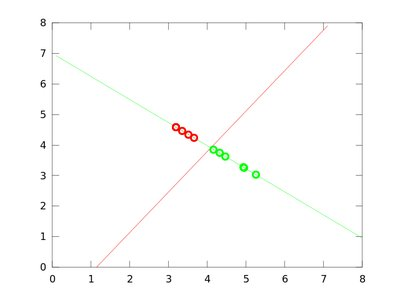While the second principal component had a smaller variance, it provided a much better discrimination between the two classes. Let's see how we can extract such a feature.

### Linear Discriminant Analysis

What we aim for is a projection, that maintains the maximum discriminative power of a given dataset, so a method should make use of class labels (if they are known a priori).
The Linear Discriminant Analysis, invented by R. A. Fisher (1936), does so by maximizing the between-class scatter, while minimizing the within-class scatter at the same time.

I took the equations from Ricardo Gutierrez-Osuna's: Lecture notes on Linear Discriminant Analysis and Wikipedia on LDA.

We'll use the same data as for the PCA example. Again create the data in `X` with corresponding classes in `c`:

```X = [2 3;3 4;4 5;5 6;5 7;2 1;3 2;4 2;4 3;6 4;7 6];
c = [  1;  1;  1;  1;  1;  2;  2;  2;  2;  2;  2];
```

Again find the indices of each class {1,2}:

```c1 = X(find(c==1),:)
c2 = X(find(c==2),:)
```

And then plot the data:

```figure;

p1 = plot(c1(:,1), c1(:,2), "ro", "markersize",10, "linewidth", 3); hold on;
p2 = plot(c2(:,1), c2(:,2), "go", "markersize",10, "linewidth", 3)

xlim([0 8])
ylim([0 8])
```

So how does a Linear Discriminant Analysis perform on this dataset?

#### Finding the projection matrix

Finding the between and within-classes scatter matrices is easy for our two classes example:

```classes = max(c)
mu_total = mean(X)
mu = [ mean(c1); mean(c2) ]
Sw = (X - mu(c,:))'*(X - mu(c,:))
Sb = (ones(classes,1) * mu_total - mu)' * (ones(classes,1) * mu_total - mu)
```

The General Eigenvalue problem is now solved by simply doing:

```[V, D] = eig(Sw\Sb)
```

And that's it. Now let's see what separations the LDA method has found! Don't forget to first sort the Eigenvectors in a descending order:

```% sort eigenvectors desc
[D, i] = sort(diag(D), 'descend');
V = V(:,i);
```

and then plot them:

```scale = 5
pc1 = line([mu_total(1) - scale * V(1,1) mu_total(1) + scale * V(1,1)], [mu_total(2) - scale * V(2,1) mu_total(2) + scale * V(2,1)]);

set(pc1, 'color', [1 0 0], "linestyle", "--")
```

Let's look at the component found by the LDA: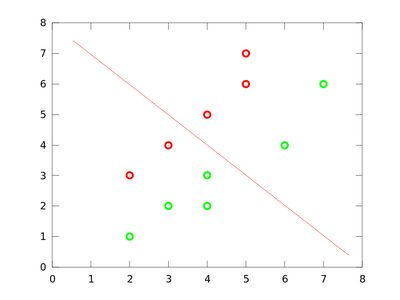Yeah! Perfect separation on the first component, that was what we were looking for!

#### projection and reconstruction

First shift the data to the new center:

```Xm = bsxfun(@minus, X, mu_total)
```

then calculate the projection and reconstruction:

```z = Xm*V(:,1)
% and reconstruct it
p = z*V(:,1)'
p = bsxfun(@plus, p, mu_total)
```

and now plotting it:

```% delete old plots
delete(p1);delete(p2);

y1 = p(find(c==1),:)
y2 = p(find(c==2),:)

p1 = plot(y1(:,1),y1(:,2),"ro", "markersize", 10, "linewidth", 3);
p2 = plot(y2(:,1), y2(:,2),"go", "markersize", 10, "linewidth", 3);
```

yields this beautiful plot:## Experiment 2: Wine Dataset

The first experiment was somewhat constructed. Let's get our hands now on some real data from the UCI Machine Learning Repository. I decided to test both methods on the Wine Dataset, an admittedly easy dataset. It consists of 178 samples with 13 constituents drawn from three types of wine.

### Functions

First of all we will turn the methods from the first experiment into functions to make them a bit more usable:

```function Z = zscore(X)
Z = bsxfun(@rdivide, bsxfun(@minus, X, mean(X)), std(X));
end

function [D, W_pca] = pca(X)
mu = mean(X);
Xm = bsxfun(@minus, X ,mu);
C = cov(Xm);
[W_pca,D] = eig(C);
[D, i] = sort(diag(D), 'descend');
W_pca = W_pca(:,i);
end

function [D, W_lda] = lda(X,y)
dimension = columns(X);
labels = unique(y);
C = length(labels);
Sw = zeros(dimension,dimension);
Sb = zeros(dimension,dimension);
mu = mean(X);

for i = 1:C
Xi = X(find(y == labels(i)),:);
n = rows(Xi);
mu_i = mean(Xi);
XMi = bsxfun(@minus, Xi, mu_i);
Sw = Sw + (XMi' * XMi );
MiM =  mu_i - mu;
Sb = Sb + n * MiM' * MiM;
endfor

[W_lda, D] = eig(Sw\Sb);
[D, i] = sort(diag(D), 'descend');
W_lda = W_lda(:,i);
end

function X_proj = project(X, W)
X_proj = X*W;
end

function X = reconstruct(X_proj, W)
X = X_proj * W';
end
```

Loading the Wine Dataset is easy in GNU Octave with the `dlmread` function. I am sure there is a similar function in MATLAB:

```% http://archive.ics.uci.edu/ml/datasets/Wine
y = data(:,1);
X = data(:,2:end);
```

Everything's ok?

```> size(X)
ans =

178    13

> size(y)
ans =

178     1

> length(unique(y))
ans = 3
```

Everything was loaded correctly. 178 instances with 13 attributes from 3 classes.

### Principal Components without normalization

With the functions it's easy to perform a PCA:

```[DPca, Wpca] = pca(X);
Xm = bsxfun(@minus, X, mean(X));
Xproj = project(Xm, Wpca(:,1:2));
```

According to the eigenvalues, the first component already accounts for 99% of the total variance.

```> cumsum(DPca) / sum(DPca)
ans =

0.99809
0.99983
0.99992
0.99997
0.99998
0.99999
1.00000
1.00000
1.00000
1.00000
1.00000
1.00000
1.00000
```

But the plot

```wine1 = Xproj(find(y==1),:);
wine2 = Xproj(find(y==2),:);
wine3 = Xproj(find(y==3),:);

figure;
plot(wine1(:,1), wine1(:,2),"ro", "markersize", 10, "linewidth", 3); hold on;
plot(wine2(:,1), wine2(:,2),"go", "markersize", 10, "linewidth", 3);
plot(wine3(:,1), wine3(:,2),"bo", "markersize", 10, "linewidth", 3);
title("PCA (original data)")
```

shows that the clusters are not well separated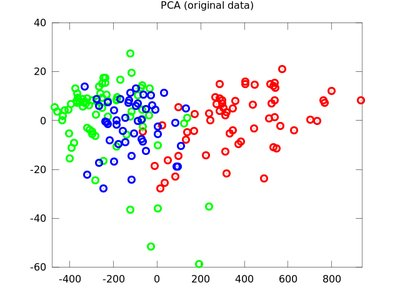### Linear Discriminant Analysis without normalization

If we apply Fisher's Discriminant Analysis on the Wine Dataset:

```[D, W_lda] = lda(X,y);
Xproj = project(Xm, W_lda(:,1:2));
```

and project it on the first two components, the classes are way better separated: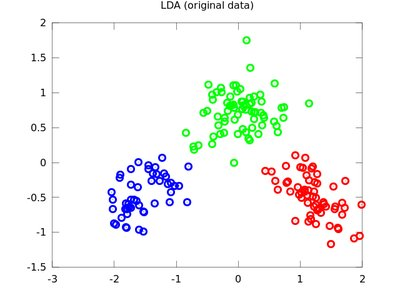### When normalization matters

The PCA performs bad. Why is that? Because the features are all on a different scale. A common trick is to scale the input to zero mean and unit variance, also called z-scores. If the normalization is applied on the data:

```Xm = zscore(X);
```

then the first principal component is responsible for only 36% of the total variance. We have to take 8 components to rise above 90% variance.

```> cumsum(DPca) / sum(DPca)
ans =

0.36199
0.55406
0.66530
0.73599
0.80162
0.85098
0.89337
0.92018
0.94240
0.96170
0.97907
0.99205
1.00000
```

On normalized data the clusters are much better separated by the PCA:So keep in mind to normalize your data sometimes.

## Appendix

### R

If you don't like GNU Octave for some reasons, you can also use R for the above examples. Please note that there is already the sophisticated function princomp in R, so you shouldn't really do it by yourself. Anyway, here is the first example translated to R. Please note I am by no means an R expert:

```repmat <- function(data, nrows, ncols, byrow=T) {
ncols <- length(data) * ncols
rep <- matrix(rep(data, nrows), nrow=nrows, ncol=ncols, byrow=byrow)
return(rep)
}

data <- c(2,3,3,4,4,5,5,6,5,7,2,1,3,2,4,2,4,3,6,4,7,6)
y <- c(1,1,1,1,1,2,2,2,2,2,2);
X <- matrix(data, ncol=2, byrow=T)
mean <- colMeans(X)
Xm <- X - repmat(mean, nrow(X), 1)
C <- cov(Xm)
eig <- eigen(C)

z <- Xm%*%eig\$vectors[,1]
p <- z%*%eig\$vectors[,1]
p <- p + repmat(mean, nrow(p), 1)

plot(p[y==1,], col="red", xlim=c(0,8), ylim=c(0,8))
points(p[y==2,], col="green")

title(main="PCA projection", xlab="X", ylab="Y")
```